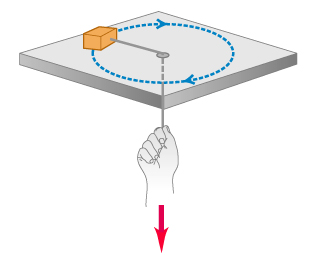# Problem: A small block with a mass of 0.0600 kg is attached to a cord passing through a hole in a frictionless, horizontal surface . The block is originally revolving at a distance of 0.41 m from the hole with a speed of 0.71 m/s . The cord is then pulled from below, shortening the radius of the circle in which the block revolves to 0.15 m . At this new distance, the speed of the block is 1.94 m/s .What is the tension in the cord in the original situation when the block has speed exttip{v_{ m 0}}{v_0} = 0.71 m/s ?What is the tension in the cord in the final situation when the block has speed exttip{v_{ m 1}}{v_1} = 1.94 m/s ?How much work was done by the person who pulled on the cord?

⚠️Our tutors found the solution shown to be helpful for the problem you're searching for. We don't have the exact solution yet.

###### Problem Details

A small block with a mass of 0.0600 kg is attached to a cord passing through a hole in a frictionless, horizontal surface. The block is originally revolving at a distance of 0.41 m from the hole with a speed of 0.71 m/s . The cord is then pulled from below, shortening the radius of the circle in which the block revolves to 0.15 m . At this new distance, the speed of the block is 1.94 m/s .

What is the tension in the cord in the original situation when the block has speed = 0.71 m/s ?

What is the tension in the cord in the final situation when the block has speed = 1.94 m/s ?

How much work was done by the person who pulled on the cord?